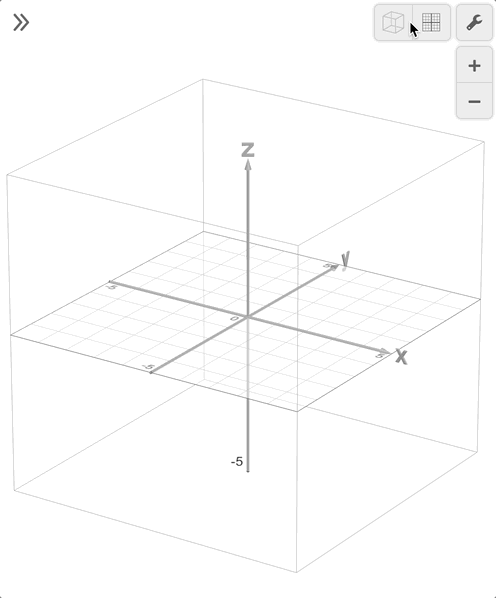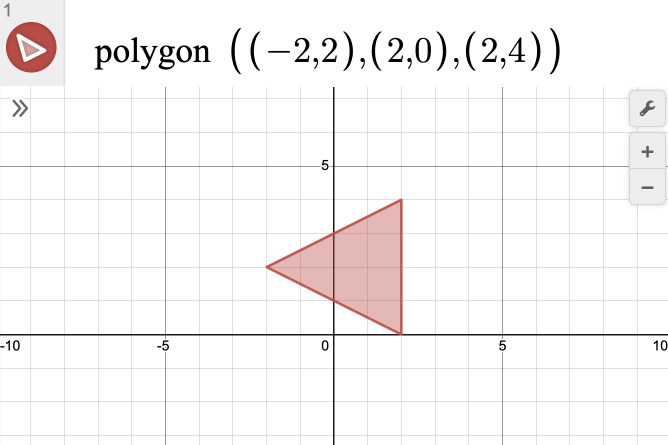# Extending from 2D to 3D

The Desmos 3D Calculator allows you to explore points, functions, and surfaces with an interface that will be familiar to users of our 2D Graphing Calculator. As you start exploring, you will encounter the same expression list for typing your equations and many familiar settings. However, you’ll also discover some new options and extensions on previous features.

### Getting Oriented

When working in 2D, your view is restricted to the $$XY$$ plane. While the default view in our 3D calculator is a cube containing the $$x$$, $$y$$, and $$z$$ axes, you can view the same 2D plane by clicking the XY Orientation button, which will animate the cube turning to snap to the $$XY$$ plane. To get back to the 3D view, you can click the Default Orientation button.### Plotting Points

One of the first things you can create in the calculator is points. When working in 2D, points are plotted as coordinate pairs of $$(x,y)$$. You can still plot an $$(x,y)$$ point in the 3D Calculator, and it will appear on the $$XY$$ plane. By adding a $$z$$ component, with coordinates of the form $$(x,y,z)$$, you have the ability to plot your point anywhere in 3D space. You can plot your points individually on an expression line, on the same line separated by commas, or with variables defined by tables, sliders, or lists.

### Extending to 3D

Equations when working in 2D are defined with the variables $$x$$ and $$y$$, whereas 3D enables you to work with expressions in $$x$$, $$y$$, and $$z$$. If you type an expression in the 3D Calculator with only $$x$$ and $$y$$ variables, you will see your curve graphed flat on the $$XY$$ plane, and a new option, labeled 'Extend to 3D,' appears below your expression, unchecked. By checking this box, you can see the entire surface of points that satisfies your expression for all $$z$$ values. Whenever your expression includes a $$z$$ variable, you won’t see this checkbox option.

### Polygons and Triangles

It is often convenient to define a surface by points rather than an explicit equation. In 2D, this is accomplished with the 'polygon' function, and in 3D, you can use the 'triangle' function. Note that while 'polygon' in 2D can take any number of points, 'triangle' in 3D can only accept 3 points as inputs.### Inequalities

In 2D, inequalities are essential for producing shaded regions on the plane, using lines or curves as boundaries. By using curly brackets, you can create a system of inequalities that restricts the $$x$$ or $$y$$ values. In 3D, this notation can be extended to create surfaces and solids by combining inequalities in $$x$$, $$y$$, and $$z$$.

### Parametrics

Parametric curves in 2D are created with an ordered pair where one or both coordinates are defined in terms of the parameter $$t$$. In 3D, a similar approach gives you the option of creating parametric surfaces. To generate a surface, your expression should have three coordinates, defined in terms of the parameters $$u$$ and $$v$$.